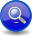#Boost C++ Libraries

...one of the most highly regarded and expertly designed C++ library projects in the world.

This is the documentation for an old version of Boost. Click here to view this page for the latest version.
##### Permutation (```a ^ b```)
###### Description

The permutation operator, ```a ^ b```, matches one or more operands (`a`, `b`, ... etc.) in any order:

```a ^ b ^ ...
```

The operands are the elements in the permutation set. Each element in the permutation set may occur at most once, but not all elements of the given set need to be present. For example:

```char_('a') ^ 'b' ^ 'c'
```

matches:

```"a", "ab", "abc", "cba", "bca" ... etc.
```
###### Header
```// forwards to <boost/spirit/home/qi/operator/permutation.hpp>
#include <boost/spirit/include/qi_permutation.hpp>
```

Also, see Include Structure.

###### Model of

Notation

`a`, `b`
###### Expression Semantics

Semantics of an expression is defined only where it differs from, or is not defined in `NaryParser`.

Expression

Semantics

```a ^ b```

Match `a` or `b` in any order. Each operand may match zero or one time as long as at least one operand matches.

###### Attributes

Expression

Attribute

```a ^ b```

```a: A, b: B --> (a ^ b): tuple<optional<A>, optional<B> >
a: A, b: Unused --> (a ^ b): optional<A>
a: Unused, b: B --> (a ^ b): optional<B>
a: Unused, b: Unused --> (a ^ b): Unused```

###### Complexity

The overall complexity of the permutation parser is defined by the sum of the complexities of its elements, s, multiplied by log s. The complexity of the permutation parser itself is O(N log N), where N is the number of elements.

###### Example
NoteThe test harness for the example(s) below is presented in the Basics Examples section.

Some using declarations:

```using boost::spirit::ascii::char_;
```

Parse a string containing DNA codes (ACTG)

```test_parser("ACTGGCTAGACT", *(char_('A') ^ 'C' ^ 'T' ^ 'G'));
```

 Copyright © 2001-2009 Joel de Guzman, Hartmut Kaiser Distributed under the Boost Software License, Version 1.0. (See accompanying file LICENSE_1_0.txt or copy at http://www.boost.org/LICENSE_1_0.txt)